you are here->home->Physics->Class 11->Boyle's Law
Boyle's Law

# Objective

To study the variation in volume with pressure for a sample of air at constant temperature by plotting graphs between P and V, and between P and 1/V.

# Theory

## Properties of gases and Gas laws

Gaseous state is a state of matter in which the substance does not have any specific shape or volume. It adopts the form and size of its container. The fundamental macroscopic properties of gases are pressure, volume, temperature and mass of the gas. These can be explained by kinetic theory by considering their molecular composition and motion.  Careful, scientific observation has determined that these variables are related to one another, and the values of these properties determine the state of the gas.

These relationships among pressure, temperature and volume of a gas lead to Gas laws. Boyle's Law tells us that the volume of gas increases as the pressure decreases. Charles' Law tells us that the volume of gas increases as the temperature increases and Avogadro's Law tells us that the volume of gas increases as the amount of gas increases. The ideal gas law is the combination of the three simple gas laws.

### Boyle’s Law

According to Boyle’s Law, the pressure (P) of a given mass of gas is inversely proportional to its volume (V), provided that the temperature of the gas remains constant.

For an enclosed gas, at constant temperature (T);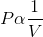or,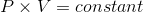ie;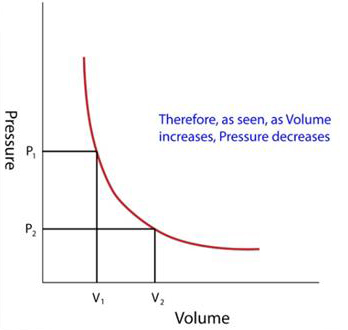The quill tube is helpful in verifying Boyle’s law.

Since the volume of gas inside the tube(V) = cross sectional area of the tube(a) × length of air column(l),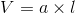So,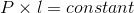# Learning outcomes

• Students learn about the properties of gases and their relationships.
• Students understand Boyles law and its applications.
• Students understand the relation between pressure and volume of a given mass of the gas.